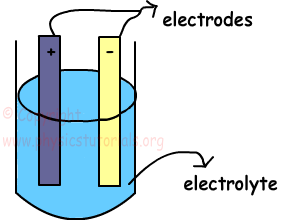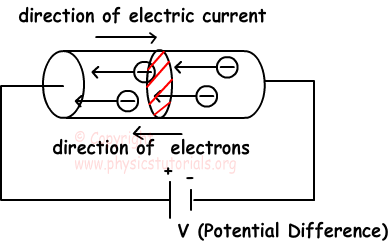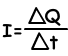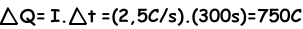Electric Current and flow of Charge

Electric BatteryElectric battery is a device that converts chemical energy to the electrical energy. It consists of two different metal plates and we call them as electrodes. One of them is negatively charged electrode and other one is positively charged cathode. These plates are placed into a solution like dilute acid and it is also called electrolyte. This set up is called electric cell and connection of electric cells produce battery. There must be a potential difference between the electrodes to make charges flow.

Electric Current and Flow of Charge

If we connect the conductor to a battery, potential difference between the ends of the conductor occurs. This potential difference creates an electric field towards to the positive end of the conductor to the negative end. Free charges inside this electric field are exerted a force F=q.E in this field. Under the effects of this force electrical charges starts to flow. This flow of charge is called electric current. If there is no potential difference then there won’t be flow of charge or electric current. We can make an analogy with heat transfer. As we discussed in earlier chapters, heat flows from matter having higher temperature to the lower temperature. In this case charges flows from higher potential to the lower potential.Electric current, in solids transferred with the free electrons, in liquids with free ions and in gases with free electrons and free ions. We can also define electric current as the charge per unit time passing through the cross section of conductor like given in the picture which is shown with red dashed lines. Average current is found with the following formula;Where; I is the current, Q is the charge and t is the time

The unit of electric current is Coulomb per second, and we give specific name Ampere (A).

1A=1C/s

Example: If the steady current 2,5 A flows in a wire for 5 minutes, find the charged passed in any point in the circuit.Electric Current Exams and Solutions

Author: